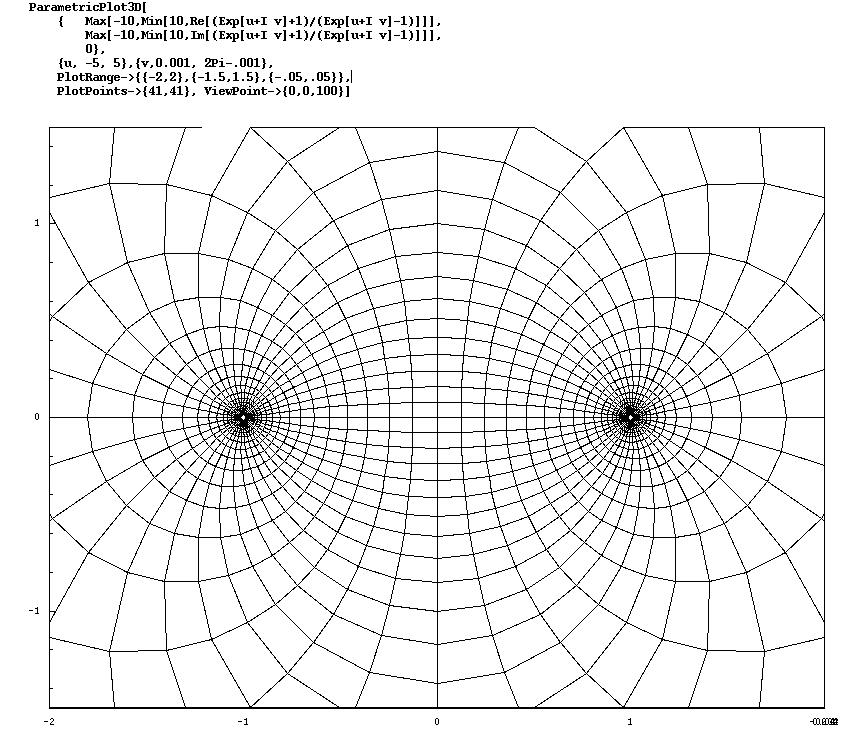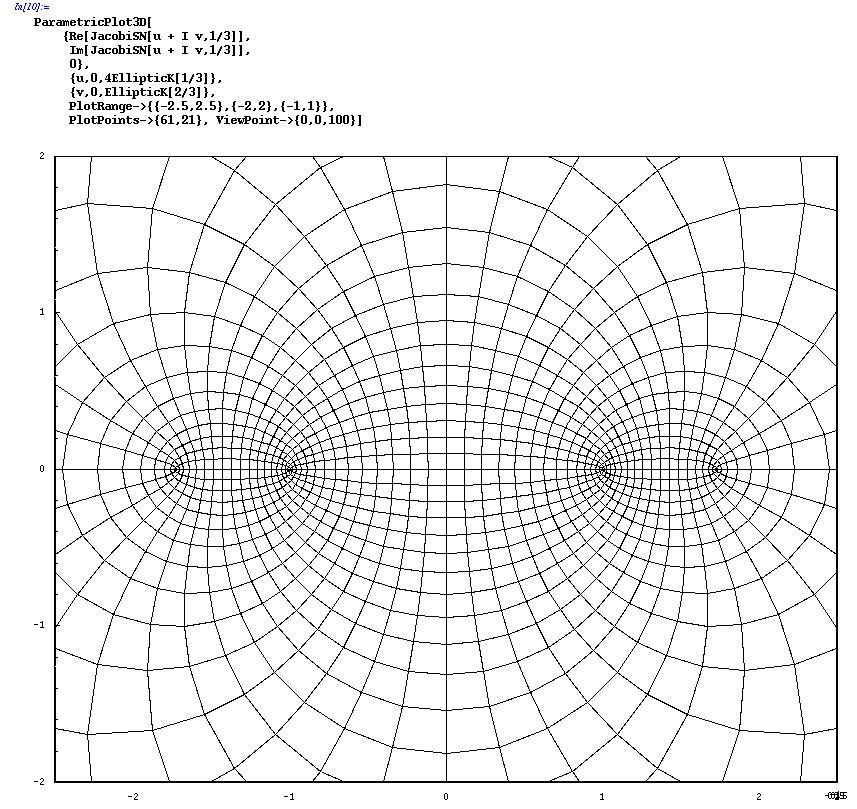# Riemann Surfaces

A Riemann surface is a 2-manifold with a complex structure (i.e. locally it looks like a piece of the complex plane). Intuitively, a Riemann surface is a sphere with handles and punctures. For example, a cylinder is topologically a sphere with two punctures, and a torus (a donut) is a sphere with one handle.

An interesting mathematical problem is to build a geometry on a given topological space. For 2-dimensional manifolds this problem is well understood - "most" 2-manifolds carry a hyperbolic geometry - but for 3-manifolds this is a subject of current research.

Philosophically, all this speaks toward the topic of "the shape of space". Is the universe infinite or finite, does it have a boundary, can it have finite volume but no boundary, is it flat or curved, what happens at infinity and beyond. Historically, the development of geometry is thought to have been motivated by a desire to model the "real" world. For centuries it was believed that Euclidean geometry was an absolute geometry that described the universe. But this idea came into question in the 1800's when it gradually became apparent that other geometries were logically possible. And this eroding of the idea of a "true" geometry in mathematics paved the way for Einstein's view of locally non-Euclidean space-time in the early 1900's.

The items on this page are an attempt to illuminate how the geometries on various Riemann surfaces arise. Riemann surfaces can be conformally mapped to the complex plane (albeit with much distortion), and it is interesting to see their geometries expressed on the plane.

## Riemann surfaces: the Cylinder

The cylinder mapped to the Complex Plane, using Mathematica:## Riemann surfaces: the Torus

The torus mapped to the Complex Plane (a 2-fold branched covering), using Mathematica